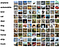# Fake News Classifier

building a classifier to detect fake news

## Objective : — Given a news article, classify whether its true or fake news

Dataset — i have used this dataset.

so let’s start.

lets import the dataset

Load the dataset

# Sentiment Analysis using Naive Bayes Algorithm

to predict a movie review whether its positive or negative.

NOTE : — updating.

# Concept of Overfitting

Demonstration of overfitting using an Polynomial Linear Regression example

Objective

To understand the concept of Overfitting using Linear Regression with Polynomial Features.

So let’s first understand What is Regression?

Have you ever thought of How can we predict price of a house or a car using Machine Learning ? Well, Regression technique is used.

Regression is used to predict a continuous value. Some of the commons Regression techniques are -

1. Simple Linear Regression

2. Multiple Linear Regression

3. Polynomial Linear Regression

Now let’s understand what is Overfitting briefly.

Let’s suppose we have a created a model & we want…

# IMAGE CLASSIFIER on CIFER10 dataset

CIFER-10 dataset exploration & experiments

Problem Statement — To build a model to classify an image. Given dataset is CIFER-10 dataset.

Outcome — Given an image, out model will predict the class of that image.

so let’s start.

What is CIFER-10 ?

CIFER-10 is a dataset of 32*32 size colored images by CIFER, which consists 10 classes (‘airplane’, ‘automobile’, ‘bird’, ‘cat’, ‘deer’, ‘dog’, ‘frog’, ‘horse’, ‘ship’, ‘truck’).CIFER-10 data samples

Let’s see, What are the Steps to Train a model ?

We will do the following steps in order:

1. Load and normalizing the CIFAR10 training and test datasets using torchvision

2. Define a…

# Titanic Data Exploration

Titanic Survival Prediction

# Overview

This is the legendary Titanic ML competition — the best, first challenge for you to dive into ML competitions and familiarize yourself with how the Kaggle platform works.

The competition is simple: use machine learning to create a model that predicts which passengers survived the Titanic shipwreck.

# The Challenge

The sinking of the Titanic is one of the most infamous shipwrecks in history.

On April 15, 1912, during her maiden voyage, the widely considered “unsinkable” RMS Titanic sank after colliding with an iceberg. …

# Why Data Science ? WhyData Science is needed?

Need of data science & what is it?

Let’s start with a team called Analytics, So what is Analytics?.

“Analytics is the discovery, interpretation, and communication of meaningful patterns in data”.

So, what is data?

“Data is any character / information that has been gathered. It can be anything like text, numbers, pictures, sound, or video.”

Data is growing exponentially each year. According to some recent report, currently the whole world is generating roughly 2. 5 quintillion bytes, that is 2. …## Jay Prakash Thakur

A Deep Learning Researcher & Developer